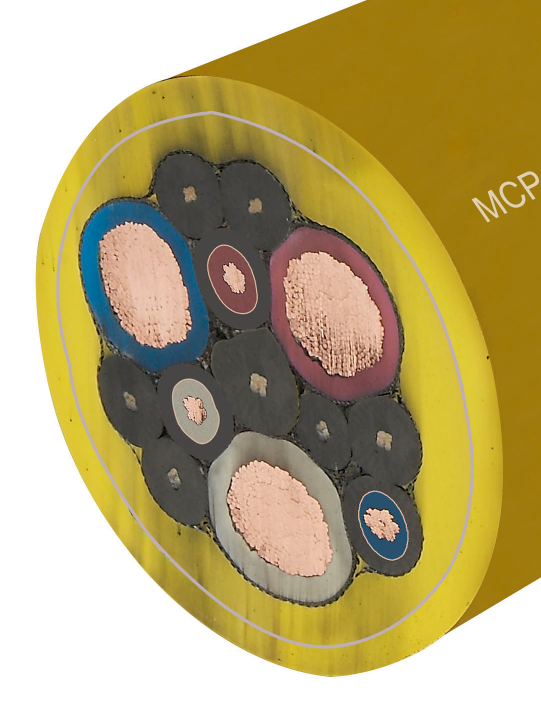# 煤矿用橡套电缆MC（UC）生产厂家发邮件给我们：872586202@qq.comMCP电缆规格

 3*16+1*4+3*2.5 3*50+1*10+7*2.5 3*25+1*6+4*2.5 3*50+1*10+3*4 3*35+1*6+4*4 3*50+1*10+4*4 3*50+1*10+4*4 3*50+1*10+3*6 3*70+1*16+3*2.5 3*50+1*10+4*6 3*95+1*25+3*2.5 3*70+1*16+3*4 3*120+1*25+3*2.5 3*70+1*16+4*4 3*25+1*6+3*2.5 3*70+1*16+3*6 3*35+1*6+3*2.5 3*70+1*16+4*6 3*35+1*6+4*2.5 3*95+1*25+3*4 3*35+1*6+3*4 3*95+1*25+4*4 3*35+1*6+4*4 3*95+1*25+3*6 3*35+1*6+3*6 3*95+1*25+4*6 3*35+1*6+4*6 3*95+1*25+3*10 3*50+1*10+3*2.5 3*95+1*25+4*10 3*50+1*10+4*2.5 3*120+1*25+3*4 3*150+1*35+3*4

天津市电缆总厂橡塑电缆厂在保证电缆绝缘性能和整体机械性能的前提下，公司根据市场需求，在橡套电缆研发的基础上自主研发了具有较强的抗电磁干扰、均匀电场、可有效消除电磁干扰，降低变频电机噪音，保证系统稳定运行的煤矿用变频装置用橡套软电缆系列新产品(MVFP)煤矿用橡套电缆MC（UC）生产厂家

### 留言框

• #### 验证码：

请输入计算结果（填写阿拉伯数字），如：三加四=7

18232657099